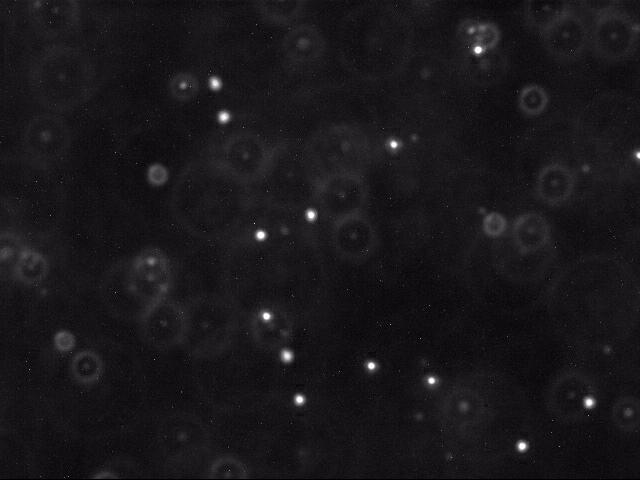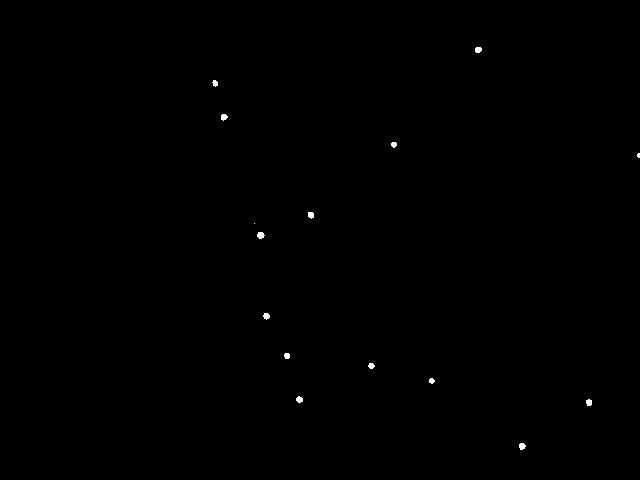COS 126 The Atomic Nature of Matter Programming Assignment Checklist

Re-affirm the atomic nature of matter by tracking the motion of particles undergoing Brownian motion, fitting this data to Einstein's model, and estimating Avogadro's number.

Historical perspective. The atom played a central role in 20th century physics and chemistry, but prior to 1908 the reality of atoms and molecules was not universally accepted. In 1827, the botanist Robert Brown observed the random erratic motion of microscopic particles suspended within the vacuoles of pollen grains. This motion would later become known as Brownian motion. Einstein hypothesized that this motion was the result of millions of even smaller particles—atoms and molecules—colliding with the larger particles.

In one of his "miraculous year" (1905) papers, Einstein formulated a quantitative theory of Brownian motion in an attempt to justify the "existence of atoms of definite finite size." His theory provided experimentalists with a method to count molecules with an ordinary microscope by observing their collective effect on a larger immersed particle. In 1908 Jean Baptiste Perrin used the recently invented ultramicroscope to experimentally validate Einstein's kinetic theory of Brownian motion, thereby providing the first direct evidence supporting the atomic nature of matter. His experiment also provided one of the earliest estimates of Avogadro's number. For this work, Perrin won the 1926 Nobel Prize in physics.

The problem. In this assignment, you will redo a version of Perrin's experiment. Your job is greatly simplified because with modern video and computer technology—in conjunction with your programming skills—it is possible to accurately measure and track the motion of an immersed particle undergoing Brownian motion. We supply video microscopy data of polystyrene spheres ("beads") suspended in water, undergoing Brownian motion. Your task is to write a program to analyze this data, determine how much each bead moves between observations, fit this data to Einstein's model, and estimate Avogadro's number.

The data.  We provide 10 datasets, obtained by William Ryu using fluorescent imaging. Each dataset is stored in a subdirectory (named run_1 through run_10) and contains a sequence of two hundred 640-by-480 color images (named frame00000.jpg through frame00199.jpg).

Here is a movie [ avi · mov ] of several beads undergoing Brownian motion. Below is a typical raw image (left) and a cleaned up version (right) using thresholding, as described below.Each image shows a two-dimensional cross section of a microscope slide. The beads move in and out of the microscope's field of view (the x- and y-directions). Beads also move in the z-direction, so they can move in and out of the microscope's depth of focus; this results in halos, and it can also result in beads completely disappearing from the image.

I. Particle identification. The first challenge is to identify the beads amidst the noisy data. Each image is 640-by-480 pixels, and each pixel is represented by a Color object which needs to be converted to a luminance value ranging from 0.0 (black) to 255.0 (white). Whiter pixels correspond to beads (foreground) and blacker pixels to water (background). We break the problem into three pieces: (i) read an image, (ii) classify the pixels as foreground or background, and (iii) find the disc-shaped clumps of foreground pixels that constitute each bead.

• Reading the image. Use the Picture data type from Section 3.1 to read the image. By convention, pixels are measured from left-to-right (x-coordinate) and top-to-bottom (y-coordinate).

• Classifying the pixels as foreground or background. We use a simple, but effective, technique known as thresholding to separate the pixels into foreground and background components: all pixels with monochrome luminance values strictly below some threshold tau are considered background; all others are considered foreground. The two pictures above illustrates the original frame (above left) and the same frame after thresholding (above right), using tau = 180.0.

• Finding the blobs. A polystyrene bead is represented by a disc-like shape of at least some minimum number min (typically 25) of connected foreground pixels. A blob or connected component is a maximal set of connected foreground pixels, regardless of its shape or size. We will refer to any blob containing at least min pixels as a bead. The center of mass of a blob (or bead) is the average of the x- and y-coordinates of its constituent pixels.

Create a helper data type Blob that has the following API.

public class Blob {
public        Blob()                     //  creates an empty blob
public   void add(int x, int y)          //  adds pixel (x, y) to this blob
public    int mass()                     //  number of pixels added to this blob
public double distanceTo(Blob that)      //  Euclidean distance between the center of masses of the two blobs
public String toString()                 //  string representation of this blob (see below)
public static void main(String[] args)   //  tests this class by directly calling all instance methods
}

String representation. The toString() method returns a string containing the blob's mass; followed by whitespace; followed by the x- and y-coordinates of the blob's center of mass, enclosed in parentheses, separated with a comma, and using four digits of precision after the decimal point.

Performance requirement. The constructor and each instance method must take constant time.

Next, write a data type BeadFinder that has the following API. Use a recursive depth-first search to identify the blobs and beads efficiently.

public class BeadFinder {
//  finds all blobs in the specified picture using luminance threshold tau
public BeadFinder(Picture picture, double tau)

//  returns all beads (blobs with >= min pixels)
public Blob[] getBeads(int min)

//  test client, as described below
public static void main(String[] args)
}

The main() method takes an integer min, a floating-point number tau, and the name of an image file as command-line arguments; create a BeadFinder object using a luminance threshold of tau; and and print all beads (blobs containing at least min pixels), as shown here:
% java-introcs BeadFinder 0 180.0 run_1/frame00001.jpg
29 (214.7241,  82.8276)
36 (223.6111, 116.6667)
1 (254.0000, 223.0000)
42 (260.2381, 234.8571)
35 (266.0286, 315.7143)
31 (286.5806, 355.4516)
37 (299.0541, 399.1351)
35 (310.5143, 214.6000)
31 (370.9355, 365.4194)
28 (393.5000, 144.2143)
27 (431.2593, 380.4074)
36 (477.8611,  49.3889)
38 (521.7105, 445.8421)
35 (588.5714, 402.1143)
13 (638.1538, 155.0000)

% java-introcs BeadFinder 25 180.0 run_1/frame00001.jpg
29 (214.7241,  82.8276)
36 (223.6111, 116.6667)
42 (260.2381, 234.8571)
35 (266.0286, 315.7143)
31 (286.5806, 355.4516)
37 (299.0541, 399.1351)
35 (310.5143, 214.6000)
31 (370.9355, 365.4194)
28 (393.5000, 144.2143)
27 (431.2593, 380.4074)
36 (477.8611,  49.3889)
38 (521.7105, 445.8421)
35 (588.5714, 402.1143)

In the sample frame, there are 15 blobs, 13 of which are beads.

II. Particle tracking. The next step is to determine how far a bead moves from one time t to the next time t + Δt. For our data, there are Δt = 0.5 seconds between frames. Assume the data is such that each bead moves a relatively small amount and that beads never collide with one another. (You must, however, account for the possibility that the bead disappears from the frame, either by departing the microscope's field of view in the x- or y- direction, or moving out of the microscope's depth of focus in the z-direction.) Thus, for each bead at time t + Δt, calculate the closest bead at time t (in Euclidean distance) and identify these two as the same bead. However, if the distance is too large—greater than delta pixels—assume that one of the beads has either just begun or ended its journey.

Write a main() method in BeadTracker.java that takes an integer min, a double value tau, a double value delta, and a sequence of image filenames as command-line arguments; identifies the beads (using the specified values for min and tau) in each image (using BeadFinder); and prints the distance that each bead moves from one frame to the next (assuming that distance is no longer than delta). You will do this for beads in each pair of consecutive frames, printing each distance that you discover, one after the other.

% java-introcs BeadTracker 25 180.0 25.0 run_1/*.jpg
7.1833
4.7932
2.1693
5.5287
5.4292
4.3962
...
Note: with this procedure, there is no need to build a data structure that tracks an individual bead through a sequence of frames.

III. Data analysis. Einstein's theory of Brownian motion connects microscopic properties (e.g., radius, diffusivity) of the beads to macroscopic properties (e.g., temperature, viscosity) of the fluid in which the beads are immersed. This amazing theory enables us to estimate Avogadro's number with an ordinary microscope by observing the collective effect of millions of water molecules on the beads.

• Estimating the self-diffusion constant. The self-diffusion constant D characterizes the stochastic movement of a molecule (bead) through a homogeneous medium (the water molecules) as a result of random thermal energy. The Einstein–Smoluchowski equation states that the random displacement of a bead in one dimension has a Gaussian distribution with mean zero and variance σ2 = 2 D Δt, where Δt is the time interval between position measurements. That is, a molecule's mean displacement is zero and its mean square displacement is proportional to the elapsed time between measurements, with the constant of proportionality 2D. We estimate σ2 by computing the variance of all observed bead displacements in the x- and y-directions. Let (Δx1, Δy1), ..., (Δxn, Δyn) be the n bead displacements, and let r1, ..., rn denote the radial displacements. Then

\begin{align} \hat \sigma^2 \;\; & = \;\; \frac{(\Delta x_1^2 + \ldots + \Delta x_n^2) \;+\; (\Delta y_1^2 + \ldots + \Delta y_n^2)}{2n} \\ \;\; & = \;\; \frac{r_1^2 + \ldots + r_n^2}{2n} \end{align}

For our data, Δt = 0.5, so $$\hat \sigma^2$$ is an estimate for D as well.

Note that the radial displacements in the formula above are measured in meters. The radial displacements output by your BeadTracker program are measured in pixels. To convert from pixels to meters, multiply by 0.175 × 10−6 (meters per pixel).

• Estimating the Boltzmann constant. The Stokes–Einstein relation asserts that the self-diffusion constant of a spherical particle immersed in a fluid is given by

$$D = \dfrac{k \, T}{6 \, \pi \, \eta \, \rho}$$
where, for our data,

• T = absolute temperature = 297 Kelvin (room temperature)

• $$\eta$$ = viscosity of water at room temperature = 9.135 × 10−4 N·s·m−2

• $$\rho$$ = radius of bead = 0.5 × 10−6 meters

and k is the Boltzmann constant. All parameters are given in SI units. The Boltzmann constant is a fundamental physical constant that relates the average kinetic energy of a molecule to its temperature. We estimate k by measuring all of the parameters in the Stokes–Einstein equation, and solving for k.

• Estimating Avogadro's number. Avogadro's number NA is defined to be the number of particles in a mole. By definition, k = R / NA, where the universal gas constant R is approximately 8.31446. Use R / k as an estimate of Avogadro's number.

For the final part, write a main() method in Avogadro.java that reads the radial displacements (r1, r2, r3, ...) from standard input and estimates Boltzmann's constant and Avogadro's number using the formulas described above.

% more displacements-run_1.txt
7.1833
4.7932
2.1693
5.5287
5.4292
4.3962
...

% java-introcs Avogadro < displacements-run_1.txt
Boltzmann = 1.2535e-23

% java-introcs BeadTracker 25 180.0 25.0 run_1/*.jpg | java-introcs Avogadro
Boltzmann = 1.2535e-23